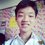# $\sqrt{m+\sqrt{n+\sqrt{m+\sqrt{n+\sqrt{m+\sqrt{n+...}}}}}}=6$

Let's solve a fun problem relating infinite square roots,

Find the number of pairs of positive integers (m, n) such that $\sqrt{m+\sqrt{n+\sqrt{m+\sqrt{n+\sqrt{m+\sqrt{n+...}}}}}}=6$ .

First, we observe that

$\sqrt{m+\sqrt{n+\sqrt{m+\sqrt{n+\sqrt{m+\sqrt{n+...}}}}}}=6$ is equal to

$\sqrt{m+\sqrt{n+6}}=6$ since the expression is infinite, and we can ONLY DO THIS IFF IT'S INFINITE, take note. So, the problem reduces to find the number of pairs of positive integers $(m,n)$ that satisfies the equation,

$\sqrt{m+\sqrt{n+6}}=6$

We can first say that $m<36$ since $\sqrt{n+6}$ and $n$ are positive.

Let's square both sides and simplify the expression a little bit, and at last it will become

$m^2-72m-n+1290=0$ and it's a quadratic equation in $m$ which has real solutions if the discriminant, $\Delta \ge 0$.

$\Delta = 72^2-4(1)(-n+1290) \ge 0$

$\Rightarrow 24+4n \ge 0$

$\Rightarrow n \ge -6$ which is trivial since $n>0$.

Also, using the quadratic formula, we get,

$m=\frac{72\pm\sqrt{24+4n}}{2}=36\pm\sqrt{6+n}$ since $m<36$ we shall take the negative sign, this leaves

$m=36-\sqrt{6+n}$ . Thus, let $\sqrt{6+n}=k <36$, where $k$ is a positive integer, then $n=k^2-6$.

For $n,m>0$, $k=3,4,5,...,35$, at last, there are $33$ pairs of positive integers $(m,n)$.Note by ChengYiin Ong
1 year, 8 months ago

This discussion board is a place to discuss our Daily Challenges and the math and science related to those challenges. Explanations are more than just a solution — they should explain the steps and thinking strategies that you used to obtain the solution. Comments should further the discussion of math and science.

When posting on Brilliant:

• Use the emojis to react to an explanation, whether you're congratulating a job well done , or just really confused .
• Ask specific questions about the challenge or the steps in somebody's explanation. Well-posed questions can add a lot to the discussion, but posting "I don't understand!" doesn't help anyone.
• Try to contribute something new to the discussion, whether it is an extension, generalization or other idea related to the challenge.

MarkdownAppears as
*italics* or _italics_ italics
**bold** or __bold__ bold
- bulleted- list
• bulleted
• list
1. numbered2. list
1. numbered
2. list
Note: you must add a full line of space before and after lists for them to show up correctly
paragraph 1paragraph 2

paragraph 1

paragraph 2

[example link](https://brilliant.org)example link
> This is a quote
This is a quote
    # I indented these lines
# 4 spaces, and now they show
# up as a code block.

print "hello world"
# I indented these lines
# 4 spaces, and now they show
# up as a code block.

print "hello world"
MathAppears as
Remember to wrap math in $$ ... $$ or $ ... $ to ensure proper formatting.
2 \times 3 $2 \times 3$
2^{34} $2^{34}$
a_{i-1} $a_{i-1}$
\frac{2}{3} $\frac{2}{3}$
\sqrt{2} $\sqrt{2}$
\sum_{i=1}^3 $\sum_{i=1}^3$
\sin \theta $\sin \theta$
\boxed{123} $\boxed{123}$

Sort by:

Great. Thanks 😊

- 1 year, 8 months ago